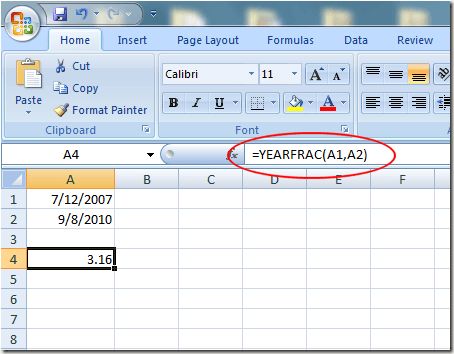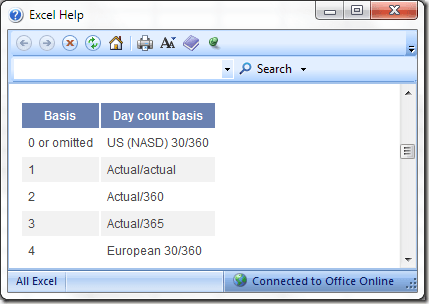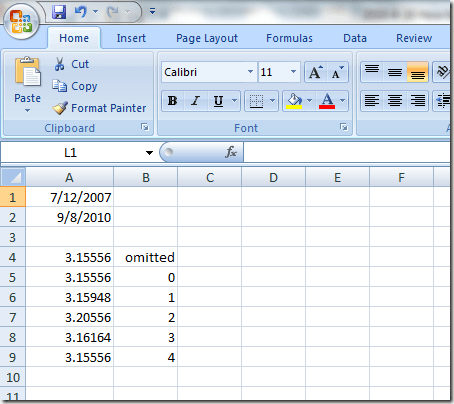One of the limitations of subtracting dates in Excel is that the application can only give you either the number of days, the number of months, or the number of years separately, rather than a combined number.

Luckily, Microsoft included a built in Excel function to give you precise differences between two dates in a worksheet. Learn how to calculate the amount of time between two dates with precision in Excel.

## Using the YEARFRAC function in Excel

Using the YEARFRAC function, you can calculate the precise difference between two dates because unlike other methods that return an integer result, this function returns a decimal result to indicate fractions of a year.

The YEARFRAC function, however, requires a bit more knowledge than most other functions. The basic formula for using this function is:

`=YEARFRAC(start_date, end_date, basis)`

Start_date is the first variable date, end_date is the second variable date, and basis is the assumption under which Excel should return the result of the calculation. It’s the basis with which you must be careful when using the YEARFRAC function.

Suppose you have an Excel worksheet that looks something like this and you want to calculate the precise difference between the two dates in A1 and A2:Rounded to two decimal places, Excel returned a result of 3.16 years using the YEARFRAC function. However, because we did not include the basis variable in the equation, Excel assumed that there are exactly 30 days in every month giving a total year’s length of just 360 days.

There are five values that you can use for the basis variable, each corresponding to a different assumption about the length of one year.According to the help document, either omitting or using a value of 0 for the basis variable forces Excel to assume the US NASD standard of 30-day months and 360-day years.

This may seem strange until you realize that many financial calculations are made under these assumptions. All of the possible values for the basis variable include:

• `0 – US NASD 30 day months/360 day years`
• `1 – Actual days in the months/Actual days in the years`
• `2 – Actual days in the months/360 days in the years`
• `3 – Actual days in the months/365 days in the years`
• `4 – European 30 days in the months/360 days in the years`

Notice that the value for the basis variable that will return the most accurate number between two dates is 1. Below are the results of using each of the values for the basis variable:Although some of the values for the basis variable may seem strange, different combinations of assumptions about the length of one month and one year are used in several fields such as economics, finance, and operations management.

In order to remain comparable between months with different numbers of days (think February vs. March) and between years with different numbers of days (think leap years vs. calendar years), these professions often make strange assumptions that the average person would not.

Particularly useful to the financier is using the assumptions offered by the basis variable to calculate APRs and APYs based on different interest compounding scenarios. Interest can be calculated continuously, daily, weekly, monthly, yearly or even span multiple years.

With the assumptions built into the YEARFRAC function, you can be sure your calculations are accurate and comparable with other calculations using the same assumptions.

As stated above, a value of 1 for the basis variable is technically the most accurate. If in doubt, choose 1 unless you are certain of the assumptions you want to make regarding the length of a month and a year. Enjoy!